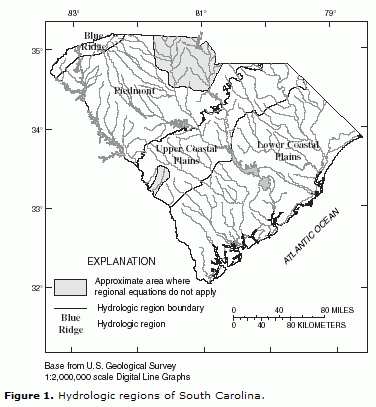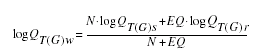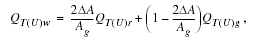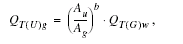Fact Sheet 001-00

# The National Flood-Frequency Program—Methods for Estimating Flood Magnitude and Frequency in Rural and Urban Areas in South Carolina, 2000

U.S. Geological Survey Fact Sheet 001-00 (Published 2000)

By Robert R. Mason, Jr.; Luis A. Fuste; Jeffrey N. King; Wilbert O. Thomas, Jr.This report is available online in pdf format (1 MB): FS 001-00.pdf

## IntroductionEstimates of the magnitude and frequency of flood-peak discharges and flood hydrographs are used for a variety of purposes, such as for the design of bridges, culverts, and flood-control structures; and for the management and regulation of flood plains. To provide simple methods of estimating flood-peak discharges, the U.S. Geological Survey (USGS) has developed and published equations for every State, the Commonwealth of Puerto Rico, American Samoa and a number of metropolitan areas in the United States. In 1993, the USGS, in cooperation with the Federal Emergency Management Agency and the Federal Highway Administration, compiled all current USGS statewide and metropolitan area equations into a computer program, titled The National Flood-Frequency (NFF) Program (Jennings and others, 1994).

Since 1993, new or updated equations have been developed by the USGS for various areas of the Nation. These new equations have been incorporated into an updated version of the NFF Program.

This Fact Sheet describes the application of the updated NFF Program to streams that drain rural and urban areas in South Carolina. Information on obtaining the NFF software and fact sheets for other areas of the Nation is provided at the end of this Fact Sheet.

## Overview

Guimaraes and Bohman (1992) developed regression equations for estimating peak discharges (QT), in cubic feet per second, that have recurrence intervals (T) that range from 2 to 500 years for unregulated, nontidal, rural streams in South Carolina. Separate sets of equations were developed for each of four hydrologic regions (fig. 1), which were determined on the basis of physiography, geology, soil characteristics, and analysis of geographic patterns in the differences between observed and estimated peak discharges for the stations used in the regression analyses. The regression equations were developed from peak-discharge records available through 1988 at 174 stream-gaging stations in South Carolina (52 stations) and adjacent areas of North Carolina (69 stations) and Georgia (53 stations). Guimaraes and Bohman (1992) summarized stream-gaging-station information, peak-discharge records, and peak-discharge estimates derived from the peak-discharge records for these 174 stations, and for selected sites on regulated streams in South Carolina.

The regression equations do not apply to some areas of the Upper Coastal Plain and Piedmont provinces because of distinctly different physiographic characteristics and insufficient streamflow data, respectively (fig. 1).

Bohman (1992) developed regression equations for estimating peak discharges (QT), in cubic feet per second, that have recurrence intervals (T) that range from 2 to 500 years for small, urban watersheds in South Carolina. The regression equations were based on generalized least-squares regression techniques. Synthetic peak-discharge data that resulted from calibrated rainfall-runoff model simulations using long-term rainfall and evaporation data were used for 30 sites in South Carolina and Georgia, and observed peak-discharge data were used at 4 sites in Charlotte, North Carolina to develop the equations.

Recurrence interval is the reciprocal of the annual exceedance probability and represents the average number of years between exceedances. For example, a flood-peak discharge with an exceedance probability of 0.01 has a recurrence interval of 100 years. This does not imply that the 100-year flood will be equaled or exceeded each 100 years, but that it will be equaled or exceeded on the average once every 100 years.

## Procedure for Rural Areas

The equations for both the rural and urban areas are based on the inch-pound system of units, but the NFF Program will accept and report either the inch-pound or metric system of units. Drainage area (A), in square miles, is the only explanatory basin variable that is used in the regression equations for estimating QT for rural areas. The drainage area is the total area that contributes runoff upstream of the stream site of interest, and is determined from the largest scale topographic map available.

The equations, the average standard errors of prediction, the equivalent years of record, and the range of drainage area for which the equations are applicable in rural areas are shown in table 1. The average standard error of prediction is a measure of the accuracy of a regression equation when used to estimate peak discharges for ungaged watersheds similar to those used to derive the regression equation. Errors in the QT estimates for about two-thirds of the ungaged sites will be within the given standard errors. Errors increase appreciably when the drainage area is near or beyond the range limits shown in table 1. The equivalent years of record is the number of years of streamflow record needed to achieve the same accuracy as the regression equation.

Table 1. Flood-peak discharge regression equations and associated statistics for streams that drain rural areas in South Carolina (modified from Guimaraes and Bohman, 1992)

 Regression equation Average standard error Equivalent years of record of prediction, in percent Table 1. Flood-peak discharge regression equations and associated statistics for streams that drain rural areas in South Carolina (modified from Guimaraes and Bohman, 1992) [QT, rural peak discharge for recurrence interval T, 2 to 500 years; A, drainage area; Do., ditto] Blue Ridge Q2 = 103A0.79 32 3 0.6-945 Q5 = 196A0.76 31 5 Do. Q10 = 286A0.73 30 8 Do. Q25 = 429A0.70 31 11 Do. Q50 = 558A0.69 33 12 Do. Q100 = 705A0.67 35 13 Do. Q500 = 1,150A0.63 40 15 Do. Piedmont Q2 = 127A0.66 53 1 0.1-1,430 Q5 = 211A0.64 38 4 Do. Q10 = 267A0.64 31 7 Do. Q25 = 347A0.63 29 12 Do. Q50 = 410A0.63 28 15 Do. Q100 = 474A0.63 28 18 Do. Q500 = 615A0.63 30 22 Do. Upper Coastal Plain Q2 = 25A0.74 23 5 4.4-1,720 Q5 = 44A0.72 25 8 Do. Q10 = 59A0.71 26 10 Do. Q25 = 80A0.70 29 12 Do. Q50 = 97A0.70 31 13 Do. Q100 = 116A0.69 33 14 Do. Q500 = 179A0.66 39 15 Do. Lower Coastal Plain Q2 = 56A0.63 30 5 0.6-1,252 Q5 = 111A0.61 31 7 Do. Q10 = 157A0.59 34 8 Do. Q25 = 221A0.59 40 9 Do. Q50 = 275A0.58 44 9 Do. Q100 = 335A0.58 47 10 Do. Q500 = 569A0.52 47 14 Do.

## Procedure for Urban Areas

The explanatory watershed variables used in the regression equations for estimating UT for urban areas are as follow:

Drainage area (A), in square miles, is the total area that contributes runoff upstream of the location of the stream site of interest. In urban areas runoff from subwatersheds may be diverted out of or into the drainage area of interest by stormwater sewers. The areas of such subwatersheds should be subtracted or added, as appropriate, to the drainage area of the stream site of interest to compute the effective drainage area.

Impervious Area (IA) is the percentage of the drainage area, A, that is covered by impervious surfaces and can be estimated from maps or aerial photos by use of the grid-sampling method or a planimeter, and from field reconnaissance of the basin.

Rural peak discharge (QT), in cubic feet per second, is generated by using the equations of Guimaraes and Bohman (1992) for the equivalent recurrence interval (T), shown in table 1.

The regression equations, the standard errors of prediction, and the ranges over which the explanatory watershed variables are applicable are shown in table 2. The standard error of prediction increases appreciably when any explanatory watershed variable is near or beyond the range limits.

 Regression equations Average standard error of prediction, in percent Applicable range of drainage area, in square miles Table 2. Flood-peak discharge regression equations and associated statistics for streams that drain urban basins in South Carolina (modified from Bohman, 1992) [UT, urban peak discharge for recurrence interval T, 2 to 500 years, in cubic feet per second; A, drainage area; IA, impervious area; QT, rural equivalent peak discharge in cubic feet per second; Do., ditto] U2 = 1.36A0.554IA1.241Q20.323 34.3 0.18-41 10-50 U5 = 2.58A0.544IA1.170Q50.299 31.2 Do. Do. U10 = 3.77A0.536IA1.115Q100.291 29.1 Do. Do. U25 = 5.84A0.524IA1.041Q250.284 26.8 Do. Do. U50 = 7.76A0.514IA0.987Q500.283 25.8 Do. Do. U100 = 10.4A0.506IA0.932Q1000.28 25.6 Do. Do. U500 = 18.8A0.484IA0.800Q5000.281 28.5 Do. Do.

The equations in table 2 are not intended for use with basins that have impervious areas less than 10 percent or more than 50 percent, and they are not applicable to basins with significant in-channel detention or basin storage. Urbanization (imperviousness) should be roughly homogeneous over the entire basin. If the computed urban peak discharge is less than the computed rural peak discharge, hydrologic judgement is required to determine which peak-discharge estimate should be used or if a weighted estimate should be developed.

## Improving Estimates With Gaged Data

Guimaraes and Bohman (1992) developed weighting techniques to improve estimates of peak discharge at rural gaged locations by combining the estimates derived from analysis of gage records with estimates derived from the regression equations. The weights of these two independent estimates are based on the length of the gage record in years (N) and the equivalent years of records (EQ) of the applicable regression equation. The weighted estimate of peak discharge for the gaged site is computed as:where

 QT(G)w is the weighted estimate of discharge Q for recurrence interval T at the gage location, QT(G)s is the estimate of QT derived from analysis of the systematic gage records, QT(G)r is the estimate of QT derived from application of the appropriate regression equation in table 1, N is the number of years in the gage record used to compute QT(G)s, and EQ is the equivalent years of record (table 1).

The accuracy of the weighted discharge estimate, in equivalent years of record, is equal to N + EQ. The NFF Program performs these computations.

## Ungaged Sites Near Gaged Sites on the Same Streams

Guimaraes and Bohman (1992) also showed how the weighted estimate for peak discharge at a rural gaged site can be used to improve estimates of peak discharge at a rural ungaged site on the same stream that has a drainage area that is between 50 and 150 percent of the drainage area of the gaged site. The weighted estimate for the ungaged site is computed as:where

 QT(U)w is the weighted estimate of discharge QT for recurrence interval T at the ungaged site, ΔA is the absolute value of the difference between the drainage areas of the gaged site, (Ag), and the ungaged site, (Au), |Ag - Au|, QT(U)r is the peak-flow estimate for recurrence interval T at the ungaged site derived from the applicable regional equation (table 1), QT(U)g is the peak-flow estimate for recurrence interval T at the ungaged site derived from the weighted estimate of peak discharge at the gaged site, QT(G)w, by adjusting for the effect of the difference in drainage area between the gaged site and the ungaged site. QT(U)g is computed as:where b is the exponent of drainage area from the appropriate regression equation (table 1).

This weighting method can be used to improve the estimate at the ungaged site when the drainage area at the ungaged site is within 50-150 percent of the drainage area of the gaged site. Otherwise, the estimate at the ungaged site should be based only on the appropriate regression equation from table 1.

## Sites in Transition Zones

When the drainage area of the site of interest is in more than one region, a weighted estimate of the peak discharge should be computed. The equations for the appropriate regions should be applied to the total drainage area for the site, as if the entire basin was in each region. The weighted estimate is then computed by multiplying each regional estimate against the fraction of the drainage area in that region and summing the products. The NFF Program provides an algorithm for this computation.

## References

Bohman, L.R., 1992, Determination of flood hydrographs for streams in South Carolina: Volume 2. Estimation of peak-discharge frequency, runoff volumes, and flood hydrographs for urban watersheds: U.S. Geological Survey Water-Resources Investigations Report 92-4040, 79 p.

Guimaraes, W.B., and Bohman, L.R., 1992, Techniques for estimating magnitude and frequency of floods in South Carolina, 1988: U.S. Geological Survey Water-Resources Investigations Report 91-4157, 174 p.

Jennings, M.E., Thomas, W.O., Jr., and Riggs, H.C., comps., 1994, Nationwide summary of U.S. Geological Survey regional regression equations for estimating magnitude and frequency of floods for ungaged sites, 1993: U.S. Geological Survey Water-Resources Investigations Report 94-4002, 196 p.

U.S. Geological Survey
Office of Surface Water
415 National Center
Reston, Virginia 20192
(703) 648-5301

USGS hydrologic analysis software is available for electronic retrieval through the World Wide Web (WWW) at: http://water.usgs.gov/software/ . The WWW page from which the National Flood-Frequency software and user documentation can be retrieved is http://water.usgs.gov/software/NFF/

Additional earth science information is available from the USGS through the WWW at http://www.usgs.gov/ or by calling 1-888-ASK-USGS.

## REPORT AVAILABILITYThis report is available online in pdf format (1 MB): USGS FS 001-00U.S. Department of the Interior | U.S. Geological Survey